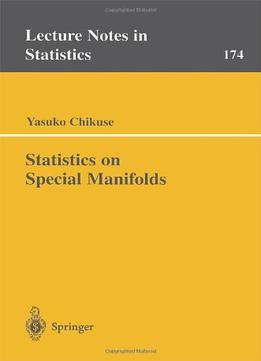Statistics On Special Manifolds (Lecture Notes In Statistics) By Yasuko Chikuse by Yasuko Chikuse / 2003 / English / PDF

Covering statistical analysis on the two special manifolds, the Stiefel manifold and the Grassmann manifold, this book is designed as a reference for both theoretical and applied statisticians. It will also be used as a textbook for a graduate course in multivariate analysis. It is assumed that the reader is familiar with the usual theory of univariate statistics and a thorough background in mathematics, in particular, knowledge of multivariate calculation techniques.

views: 379